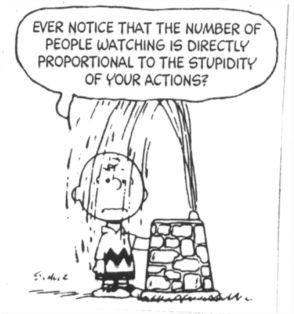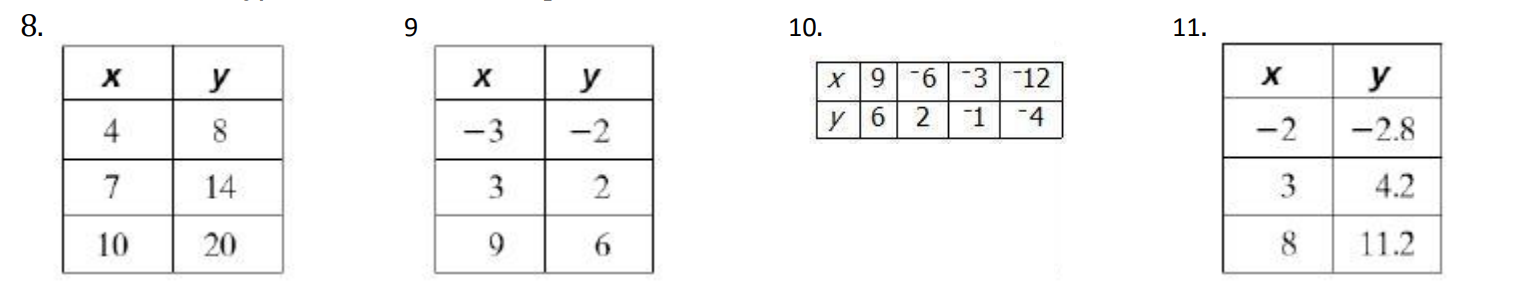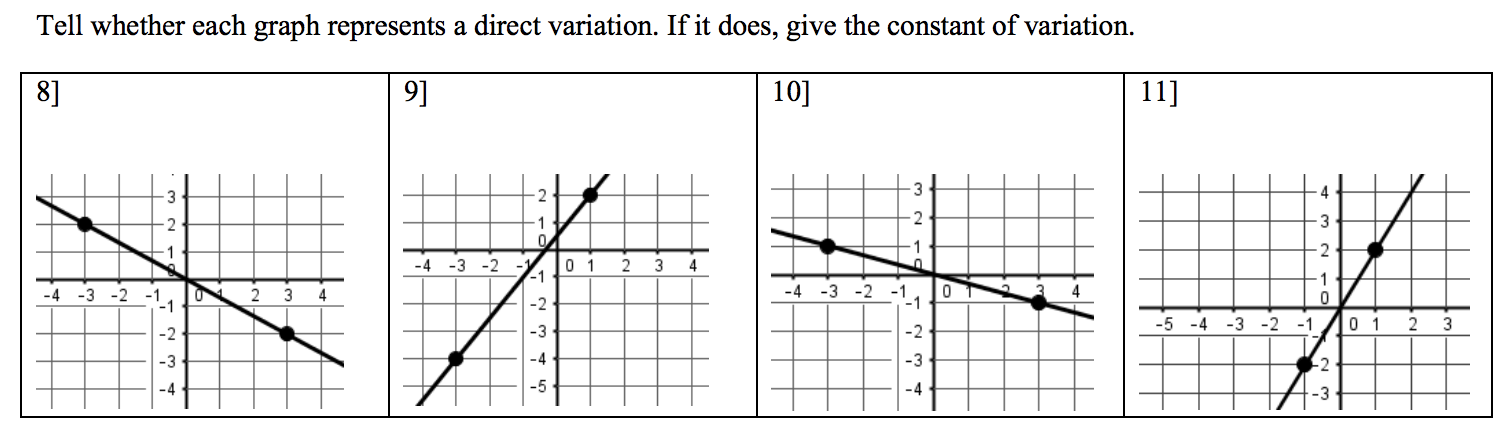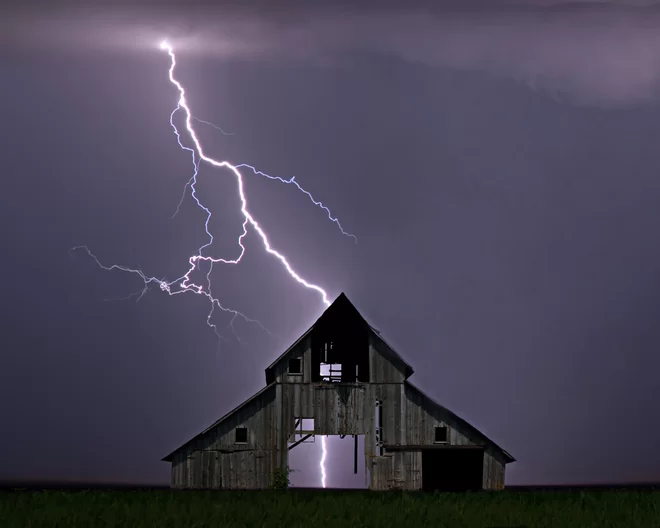Direct Variation clone
starstarstarstarstarstarstarstarstarstar
by Felix Gabathuler
| 28 Questions1
1
After watching the video, sum up what you just learned with respect to direct variation. How does it relate to slope, ratios, rates, and proportions? Write at least 3 sentences explaining your opinion.
Write the slopes of the following lines:2
3
4
2
1
3
1
4
15
6
7
8
9
10
5
1
Write yes or no for whether this equation represents direct variation. If the answer is yes, find the constant of variation (slope). Write or answer like this: yes,k=3 or no
6
1
Write yes or no for whether this equation represents direct variation. If the answer is yes, find the constant of variation (slope). Write or answer like this: yes,k=3 or no
7
1
Write yes or no for whether this equation represents direct variation. If the answer is yes, find the constant of variation (slope). Write or answer like this: yes,k=3 or no
8
1
Write yes or no for whether this equation represents direct variation. If the answer is yes, find the constant of variation (slope). Write or answer like this: yes,k=3 or no
9
1
Write yes or no for whether this equation represents direct variation. If the answer is yes, find the constant of variation (slope). Write or answer like this: yes,k=3 or no
10
1
Write yes or no for whether this equation represents direct variation. If the answer is yes, find the constant of variation (slope). Write or answer like this: yes,k=3 or no
Now let's determine if a table represents direct variation. A table is just a collection of coordinates (x,y) values that make up a line on a graph. We can tell if a table represents direct variation if the slope is the same between all the points. An easy way of testing that is to calculate if each x and y pair are proportional to each other.
If you need help deciding whether a graph represents direct variation (a proportion), watch the following video. If you feel good about it, skip it and move on.
11
1
On the graph below, draw a line that represents direct variation that passes through the point (2,3)Watch the following video to get a feel for how to solve for the k value when given x and y. It's a good video!!
12
1
What are a few key things to look for when determining if a graph represents direct variation? Name at least two.
13
1
Your distance (d) from lightning varies directly with the time (t) it takes you to hear thunder. If you hear thunder 10 seconds after you see the lightening, you are about 2 miles from the lightning.

Write a direct variation equation for the relationship between time (t) and distance (d).
14
1
Use the equation from above to determine how long it would take for the sound of thunder to travel 6 miles. Only write the number, no units.
Next, let's figure out how to solve for the constant of variation (slope). Try these three problems below. Use the x and y that are given to substitute into the y=kx equation in order to solve for k.15
16
17
18
19
20
21
22
23
24
25
26
15
1
Solve for the constant of variation using the equation y=kx. Write your answer as k=#
16
1
Write your new direct variation equation using the k you just solve for. Example answer: y=3x
17
1
Using your new direct variation, if x = -5, y = ? Write your answer like y=#
18
1
Using the same direct variation, if y = 12, x = ? Write your answer like x=#
19
1
Solve for the constant of variation using the equation y=kx. Write your answer as k=# . Remember, always reduce fractions!
20
1
Write your new direct variation equation using the k you just solve for. Example answer: y=1/4x
21
1
Using your new direct variation, if x = -3, y = ? Write your answer like y=#
22
1
Using the same direct variation, if y = -8, x = ? Write your answer like x=#
23
1
Solve for the constant of variation using the equation y=kx. Write your answer as k=# . Remember, always reduce fractions!
24
1
Write your new direct variation equation using the k you just solve for. Example answer: y=1/4x
25
1
Using your new direct variation, if x = -9, y = ? Write your answer like y=#
26
1
Using the same direct variation, if y = -8, x = ? Write your answer like x=#27
1
A recipe for 3 dozen corn muffins calls for 2 cups of flour. The number of muffins (m) varies directly with the amount of flour (f) you use.

Write a direct variation equation for the relationship between the number of cups of flour (f) and how many muffins (m) it would make.
28
1
Using the equation from above, determine how many cups of flour are needed to make 10 dozen muffins. Write your answer as a reduced improper fraction. Do not include units.